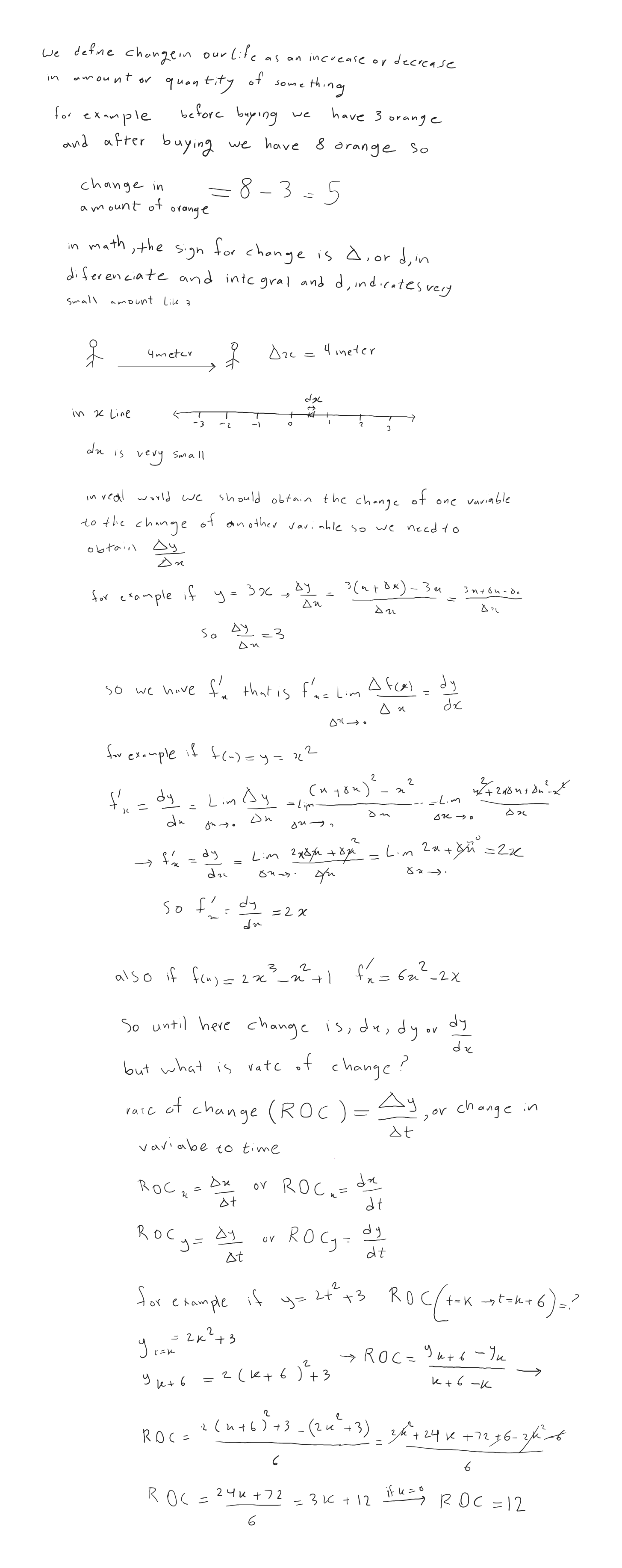SherpaMaths

>

GCSE

Rates of Change

Question

# How do you find the rate of change?

1 year ago

·

128 Replies

·

4497 views

V

Vickie Shanahan

L
Leeland

The rate of change is how something is changing. Imagine you have a toy car that moves on a track. The rate of change tells you how fast the car is moving or if it's going faster or slower.

To find the rate of change, you can look at two things: how much something changes and how much time it takes. For example, if the car moves 10 centimeters in 2 seconds, the rate of change is 5 centimeters per second because it moves 5 centimeters each second.

You can also think of the rate of change as a slope on a hill. If the hill is steep, it means things are changing quickly. If it's not very steep, things are changing slowly.

So, the rate of change is like a measurement that tells you how fast something is changing or how steep the change is. It can help you understand how things are moving or growing.

Charlotte F Verified Sherpa Tutor ✓

Cambridge graduate teaching Maths, Chemistry and Physics

To find the rate of change, in more advanced level maths than GCSE, you do something called differentiation. But this is basically like dividing your quantity by whatever it is changing by.

For example, if you had water coming out of a tap and you wanted to find the rate of flow: you would need to think about the volume (maybe in mL) that comes out per unit time (i.e. per second). So your rate of flow would be the volume divided by the time (in mL/ s). Your rate of change of flow would be your rate of flow divided by the time once more (i.e. mL/ s divided by sec again therefore mL/ s^2).

On graphs you can find rates at a certain point by drawing a tangent and finding the gradient of the tangent.

I'm available for 1:1 private online tuition!

B
Ben

To find the rate of change you simply put the change in the variable you want solved and divide by the time it took to do so e.g. velocity is the the distance travelled (starting from 0) divided by the time it took to travel (starting from 0).

H
Heet

The rate of change is simply the average of two values, dependent upon the variable. To pick the most common one, it could the output (in m^3) of a factory over time (years). To find the rate of change, we need to know its rate of growth. We can first see its ouput for the first year and the 3rd. Knowing that, we can find the change in value and divide that over the duration (2 years). Its as simple as an average measured for a specific quantity of time, or any other variable.

T
Toby Nuttall

Given a variable quantity y depending on x, we can plot a graph of y against x, and we can construct a tangent to this line at any point, the gradient of this tangent line gives the rate of change of y with respect to x at this point.

(For context a tangent line is a line who's gradient is the same as that of the curve at the specified point, which we can construct with a ruler)

F
Farooq

The rate of change (ROC) is the speed at which a variable changes over a specific period of timeF
Finn Mortimore

If we have a graph, the rate of change at a particular point is the gradient of the tangent at that point - this is known as the derivative. Given some values, we could approximate the rate of change by calculating the gradient of the graph of those values at that point i.e. rise over run

A
Aamir Zaib

you take derivative with respect to time

Think you can help?## Need a GCSE Maths tutor?

Get started with a free online introductions with an experienced and qualified online tutor on Sherpa.

Find a GCSE Maths Tutor